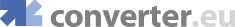online velocity and speed conversions, foot/minute, foot/second, kilometer/hour, kilometer/second
Engineering converters
Heat converters
Fluids converters
Light converters
Electricity converters
Magnetism converters
Other converters

 Show currency exchange rate: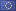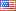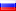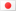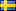… Get a Converter API tool for your website for free!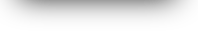All Velocity and Speed Conversion
Convert fromConvert to
ft/min =  ft/min
 Common units Foot/Minute ft/min Foot/Second ft/s Kilometer/Hour km/h Kilometer/Second km/s Knot kn Meter/Second m/s Mile/Hour mph Other units Centimeter/Hour cm/h Centimeter/Minute cm/min Centimeter/Second cm/s Foot/Hour ft/h Kilometer/Minute km/min Light Mach (At Sea Level) mach Mach (SI Standard) mach Meter/Hour m/h Meter/Minute m/min Mile/Minute mi/min Mile/Second mi/s Yard/Hour yd/h Yard/Minute yd/min Yard/Second yd/s

 Common units Foot/Minute ft/min Foot/Second ft/s Kilometer/Hour km/h Kilometer/Second km/s Knot kn Meter/Second m/s Mile/Hour mph Other units Centimeter/Hour cm/h Centimeter/Minute cm/min Centimeter/Second cm/s Foot/Hour ft/h Kilometer/Minute km/min Light Mach (At Sea Level) mach Mach (SI Standard) mach Meter/Hour m/h Meter/Minute m/min Mile/Minute mi/min Mile/Second mi/s Yard/Hour yd/h Yard/Minute yd/min Yard/Second yd/s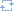Change directionMost popular convertion pairs of velocity and speed

© converter.eu  |  API  |  feedback